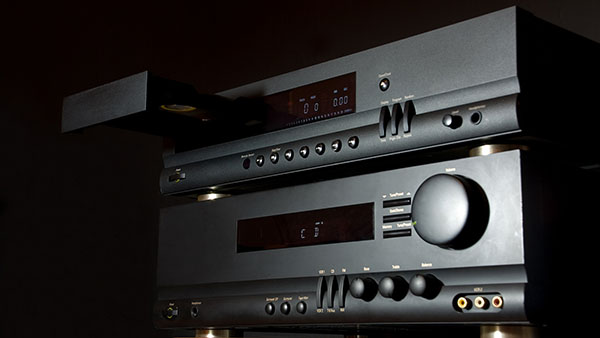# 音频编译码器

AKM的高性能、低功耗音频编译码器(CODEC)适用于各种音频系统，包括便携设备、固定设备和车载设备等。
AKM的音频编译码器不仅具备高集成度和设计自由度，而且有助于打造高清音频系统。## 产品系列

`Part Number`
```Part
Status```
```Number
of
Channels
```Number
of
Channels
(DA)```
```Bits
[bit]```
```S/N
[dB]```
```S/N
(DA)
[dB]```
```THD+N
[dB]```
```THD+N
(DA)
[dB]```
```Power
Supply
Voltage
(MIN.)
[V]```
```Power
Supply
Voltage
(TYP.)
[V]```
```Power
Supply
Voltage
(MAX.)
[V]```
```Microphone
Amplifier```
```Speaker
Amplifier```
`Other`
```Operating
Temperature
[℃]```
```Device
Package```
```Number
of Pins```
`AK4558EN`
`MP`
`2`
`2`
`32`
`108`
`108`
`-92`
`-100`
`2.4`
`3.3`
`3.6`

`PLL VELVETSOUND`
`-40 to 105`
`HWQFN`
`28`
`AK4619VN`
`MP`
`4`
`4`
```24 (AD)
32 (DA)```
`106`
`108`
`-95`
`-91`
`1.7 / 3.0`
`3.3 / 3.3`
`3.6 / 3.6`
`Yes`

`Automotive grade, TDM I/F`
`-40 to 105`
`HWQFN`
`32`
`AK4621EF`
`MP`
`2`
`2`
`24`
`115`
`115`
`-102`
`-100`
`4.75 / 3.0`
`5.0 / 3.3`
`5.25 / 3.6`

`-10 to 70`
`VSOP`
`30`
`AK4940VN`
`MP`
`1`
`1`
`24`
`90`
`90`
`-80`
`-80`
`3`

`3.6`
`Yes`

`Analog Direct Path, Automotive grade`
`-40 to 105`
`HWQFN`
`24`